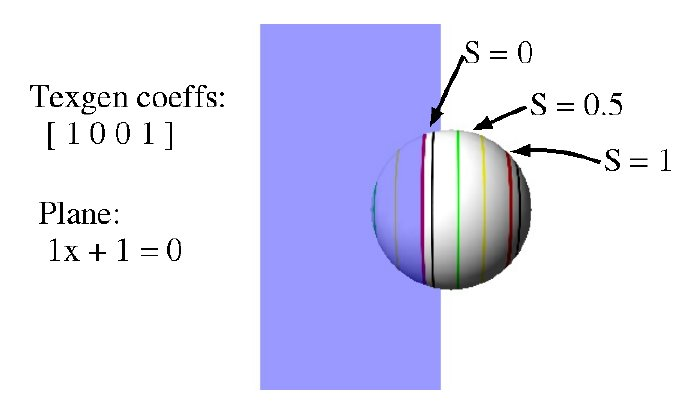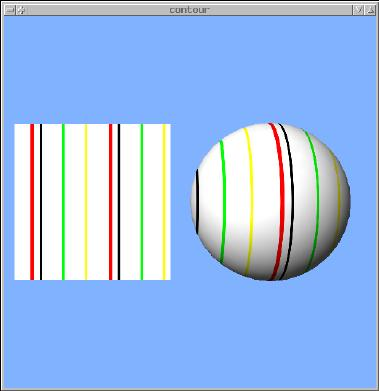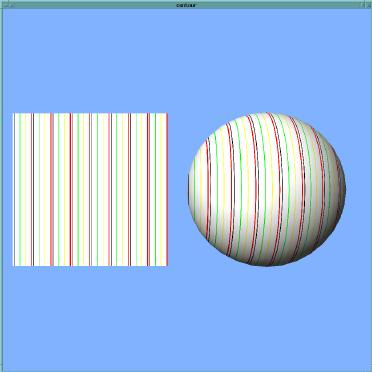# Texgen Planes

The texture generation mode can be one of

• GL_OBJECT_LINEAR
• GL_EYE_LINEAR
• GL_SPHERE_MAP

## GL_OBJECT_LINEAR

When the mode is GL_OBJECT_LINEAR, the texture coordinate is calculated using the the plane-equation coefficients that are passed via glTexGenfv(GL_S, GL_OBJECT_PLANE, planeCoefficients).

The coordinate (S in this case) is computed as:

```    S = Ax + By + Cz + D
```

using the [x y z] coordinates of the vertex (the values passed to glVertex).

If [A B C] is a unit vector, this means that the texture coordinate S is equal to the distance of the vertex from the texgen plane.We can change how frequently the texture repeats (i.e. scale the texture) by multiplying the texgen coefficients by different amounts.

e.g., changing the coefficients from [1 0 0 0] to [3.5 0 0 0] changes the results of contour.cpp from:to:## GL_EYE_LINEAR

GL_EYE_LINEAR works similarly, with the coefficients passed via glTexGenfv(GL_S, GL_EYE_PLANE, planeCoefficients).

The difference is that GL_OBJECT_LINEAR operates in "object coordinates", while GL_EYE_LINEAR works in "eye coordinates".

This means that the texture coordinates computed with GL_OBJECT_LINEAR depend solely on the values passed to glVertex, and do not change as the object (or camera) moves via transformations.

The texture coordinates computed with GL_EYE_LINEAR use the positions of vertices after all modeling & viewing transformations - i.e. they use the positions of the vertices on the screen.

Example: contourMove.cpp

next